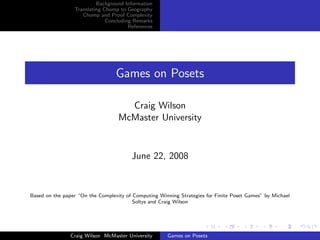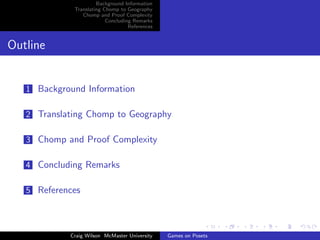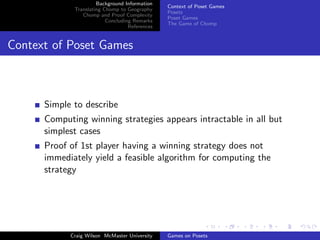Successfully reported this slideshow.

# Games on Posets - CiE 2008×

1 of 27
1 of 27

# Games on Posets - CiE 2008

## More Related Content

### Games on Posets - CiE 2008

1. 1. Background Information Translating Chomp to Geography Chomp and Proof Complexity Concluding Remarks References Games on Posets Craig Wilson McMaster University June 22, 2008 Based on the paper “On the Complexity of Computing Winning Strategies for Finite Poset Games” by Michael Soltys and Craig Wilson Craig Wilson McMaster University Games on Posets
2. 2. Background Information Translating Chomp to Geography Chomp and Proof Complexity Concluding Remarks References Outline 1 Background Information 2 Translating Chomp to Geography 3 Chomp and Proof Complexity 4 Concluding Remarks 5 References Craig Wilson McMaster University Games on Posets
3. 3. Background Information Translating Chomp to Geography Chomp and Proof Complexity Concluding Remarks References Context of Poset Games Posets Poset Games The Game of Chomp Context of Poset Games Simple to describe Computing winning strategies appears intractable in all but simplest cases Proof of 1st player having a winning strategy does not immediately yield a feasible algorithm for computing the strategy Craig Wilson McMaster University Games on Posets
4. 4. Background Information Translating Chomp to Geography Chomp and Proof Complexity Concluding Remarks References Context of Poset Games Posets Poset Games The Game of Chomp Partially Ordered Sets (Posets) Set U together with ordering relation satisﬁes following properties: Anti-Symmetry: If a b, then b a Transitivity: If a b and b c, then a c If a b and b a, then a||b (incomparable) Craig Wilson McMaster University Games on Posets
5. 5. Background Information Translating Chomp to Geography Chomp and Proof Complexity Concluding Remarks References Context of Poset Games Posets Poset Games The Game of Chomp (Finite) Poset Games  Games between 2 players From ﬁnite Poset (U, ), Poset Game (A, ) is played as follows: 1 Initialize A = U 2 Select an x ∈ A, remove all y ∈ A such that x y 3 Game ends when A = ∅, player unable to select an element loses A round is a sequence of two consecutive moves (ﬁrst player, then second) Craig Wilson McMaster University Games on Posets
6. 6. Background Information Translating Chomp to Geography Chomp and Proof Complexity Concluding Remarks References Context of Poset Games Posets Poset Games The Game of Chomp Chomp x Special case of poset game. Ordering relation can be thought of as a chocolate bar. Don’t eat the poisoned square! Craig Wilson McMaster University Games on Posets
7. 7. Background Information Translating Chomp to Geography Chomp and Proof Complexity Concluding Remarks References Context of Poset Games Posets Poset Games The Game of Chomp More Formally. . . Set of pairs (“cells”) {(i, j) |1 ≤ i ≤ n, 1 ≤ j ≤ m} Select pair (i0, j0) Remove all (i, j) such that i ≥ i0 and j ≥ j0 Player left with only (1, 1) loses Craig Wilson McMaster University Games on Posets
8. 8. Background Information Translating Chomp to Geography Chomp and Proof Complexity Concluding Remarks References Context of Poset Games Posets Poset Games The Game of Chomp Chomp Conﬁgurations Represent as binary strings X: x Figure: Chomp conﬁguration with X = 00101101 1s delimit rows, 0s indicate diﬀerence in rows lengths. Craig Wilson McMaster University Games on Posets
9. 9. Background Information Translating Chomp to Geography Chomp and Proof Complexity Concluding Remarks References Chomp ∈ PSPACE (Part 1) Geography Gadget Construction Challenges Translating Chomp to Geography Simple, direct polynomial-time translation from Poset Games to Geography, which shows Poset Games ∈ PSPACE Translation from restricted form of Geography to Chomp also attempted Limitations of poset games revealed - not PSPACE-complete? Craig Wilson McMaster University Games on Posets
10. 10. Background Information Translating Chomp to Geography Chomp and Proof Complexity Concluding Remarks References Chomp ∈ PSPACE (Part 1) Geography Gadget Construction Challenges Mathematically... GeneralizedGeography(GG): GG = { G, s | Player 1 has a winning strategy for the Generalized Geography game played on graph G starting at node s} Craig Wilson McMaster University Games on Posets
11. 11. Background Information Translating Chomp to Geography Chomp and Proof Complexity Concluding Remarks References Chomp ∈ PSPACE (Part 1) Geography Gadget Construction Challenges Graph Construction: Part 1 x yz Figure: Base Construction of a poset game in Geography Craig Wilson McMaster University Games on Posets
12. 12. Background Information Translating Chomp to Geography Chomp and Proof Complexity Concluding Remarks References Chomp ∈ PSPACE (Part 1) Geography Gadget Construction Challenges Complications... Once a node x ∈ G has been visited, must not be able to visit any y such that x y Need system of checks and balances x x1x2 y x4 x3z incoming moves incoming challenges outgoing moves Figure: 5-node gadget Craig Wilson McMaster University Games on Posets
13. 13. Background Information Translating Chomp to Geography Chomp and Proof Complexity Concluding Remarks References Chomp ∈ PSPACE (Part 1) Geography Gadget Construction Challenges Gadget Construction x x1x2 y x4 x3z incoming moves incoming challenges outgoing moves Figure: Construction of the 5-node gadget Craig Wilson McMaster University Games on Posets
14. 14. Background Information Translating Chomp to Geography Chomp and Proof Complexity Concluding Remarks References Chomp ∈ PSPACE (Part 1) Geography Gadget Construction Challenges Preventing Illegal Moves Additional nodes prevent players from making illegal moves. If player moves to node that “shouldn’t exist”, they will lose. Challenges to legal moves also result in a loss. Craig Wilson McMaster University Games on Posets
15. 15. Background Information Translating Chomp to Geography Chomp and Proof Complexity Concluding Remarks References Chomp ∈ PSPACE (Part 1) Geography Gadget Construction Challenges Example: Challenging an Illegal Move x x1x2 y x4 x3z incoming moves incoming challenges outgoing moves Figure: Challenging an illegal move Craig Wilson McMaster University Games on Posets
16. 16. Background Information Translating Chomp to Geography Chomp and Proof Complexity Concluding Remarks References The Goal Bounded Arithmetic Description of W1 1 Construction of Formula Φ(X, n, m) Existence of Winning Strategy Chomp and Proof Complexity Second proof of Chomp ∈ PSPACE Use theorems of W1 1 to show the existence of a winning strategy ∈ PSPACE Introduce segments X[i] of a conﬁguration string X: X = X 01 X 10 X 11 X 00 Craig Wilson McMaster University Games on Posets
17. 17. Background Information Translating Chomp to Geography Chomp and Proof Complexity Concluding Remarks References The Goal Bounded Arithmetic Description of W1 1 Construction of Formula Φ(X, n, m) Existence of Winning Strategy Proof Complexity Main idea: ΓC ∀x∃yα(x, y) ∃f ∈ C such that α(x, f (x)) Diﬀerent bounded arithmetic theories capture diﬀerent classes Craig Wilson McMaster University Games on Posets
18. 18. Background Information Translating Chomp to Geography Chomp and Proof Complexity Concluding Remarks References The Goal Bounded Arithmetic Description of W1 1 Construction of Formula Φ(X, n, m) Existence of Winning Strategy Variables of a Diﬀerent “Sort” Three types of variables called sorts: 1 Natural numbers (x, y, z, . . . ) 2 Strings (X, Y , Z, . . . ) 3 Sets of strings (X, Y, Z, . . . ) Concerned with formulas from class ΣB 1 : (∃X) (∀Y ) (∃Z) . . . (∀y) A (X, Y , Z, . . . , y) Craig Wilson McMaster University Games on Posets
19. 19. Background Information Translating Chomp to Geography Chomp and Proof Complexity Concluding Remarks References The Goal Bounded Arithmetic Description of W1 1 Construction of Formula Φ(X, n, m) Existence of Winning Strategy Description of W1 1  Third-sorted theory for reasoning in PSPACE Symbols taken from set L3 A = {0, 1, +, ×, ||2, ∈2, ∈3, ≤, =} Axioms : B1. x + 1 = 0 B7. (x ≤ y ∧ y ≤ x) → x = y B2. (x + 1 = y + 1) → x = y B8. x ≤ x + y B3. x + 0 = x B9. 0 ≤ x B4. x + (y + 1) = (x + y) + 1 B10. x ≤ y ∨ y ≤ x B5. x × 0 = 0 B11. x ≤ y ↔ x < y + 1 B6. x × (y + 1) = (x × y) + x B12. x = 0 → ∃y ≤ x(y + 1 = x) L1. X(y) → y < |X| L2. y + 1 = |X| → X(y) SE. [|X| = |Y | ∧ ∀i < |X|(X(i) ↔ Y (i))] → X = Y Craig Wilson McMaster University Games on Posets
20. 20. Background Information Translating Chomp to Geography Chomp and Proof Complexity Concluding Remarks References The Goal Bounded Arithmetic Description of W1 1 Construction of Formula Φ(X, n, m) Existence of Winning Strategy Achieving the Goal - Step 1 Want to give formula Φ(X, n, m) which decides whether X is valid Chomp game of size n × m X is string of length (n × m)(n + m), with (n × m) segments Φ is conjunction of three formulas: φinit, φﬁnal, and φmove Craig Wilson McMaster University Games on Posets
21. 21. Background Information Translating Chomp to Geography Chomp and Proof Complexity Concluding Remarks References The Goal Bounded Arithmetic Description of W1 1 Construction of Formula Φ(X, n, m) Existence of Winning Strategy Formulas φinit and φﬁnal φinit asserts X to be the initial conﬁguration of the game: φinit(X , n, m) = ∀i ≤ (n + m)((i > m) → X (i) = 1) φﬁnal asserts X[n×m] to be the ﬁnal conﬁguration of the game: φﬁnal(X[n×m] , n, m) = ∀i ≤ (n + m)((i > n) → X[n×m] (i) = 0) Craig Wilson McMaster University Games on Posets
22. 22. Background Information Translating Chomp to Geography Chomp and Proof Complexity Concluding Remarks References The Goal Bounded Arithmetic Description of W1 1 Construction of Formula Φ(X, n, m) Existence of Winning Strategy The φyields formula φyields asserts that each segment of X can be obtained from one legal move on the previous one: φmove(X, n, m) = ∀i < (n × m)(X[i] “yields” X[i+1] ) Deﬁning “yields” is where the fun begins! Attempt to discern what square(s) could have been played between X[i] and X[i+1] Ensure diﬀerences between conﬁgs correspond to played square Craig Wilson McMaster University Games on Posets
23. 23. Background Information Translating Chomp to Geography Chomp and Proof Complexity Concluding Remarks References The Goal Bounded Arithmetic Description of W1 1 Construction of Formula Φ(X, n, m) Existence of Winning Strategy Complete Yields Formula (∃j ≤ NumOnes)(∃k ≤ NumZeros)[F0(1, k, X) < F1(1, j, X) ∧(∃p < |X[i] |)(∃q ≤ |X[i] |)(p = F0(1, k − 1, X[i] ) + 1 ∧ q = F1(p, j, X[i] )) ∧(∀r ≤ |X|)[(r < p ∨ r > q → X[i+1] (r) = X[i] (r)) ∧(r < p + j → X[i+1] (r) = 1) ∧(r ≥ p + j → X[i+1] (r) = 0)]] Where NumOnes = |X[i] | − numones(1, X[i] ) NumZeroes = numones(1, X[i] ) Craig Wilson McMaster University Games on Posets
24. 24. Background Information Translating Chomp to Geography Chomp and Proof Complexity Concluding Remarks References The Goal Bounded Arithmetic Description of W1 1 Construction of Formula Φ(X, n, m) Existence of Winning Strategy Achieving the Goal, Step 2 Strategy function S from conﬁgurations to conﬁgurations Formula for either player having winning strategy: ∀C∃S [WinP1 (S, C) ∨ WinP2 (S, C)] where WinP1 (S, C) is a ΣB 1 formula Manipulate this to get formula for ﬁrst player having a winning strategy: (C = 0m 1n ) → ∃S [WinP1 (S, C)] Craig Wilson McMaster University Games on Posets
25. 25. Background Information Translating Chomp to Geography Chomp and Proof Complexity Concluding Remarks References The Goal Bounded Arithmetic Description of W1 1 Construction of Formula Φ(X, n, m) Existence of Winning Strategy Applying the Witnessing Theorem With W1 1 (C = 0m 1n ) → ∃S [WinP1 (S, C)] we have that the function for computing the winning strategy is in PSPACE. Craig Wilson McMaster University Games on Posets
26. 26. Background Information Translating Chomp to Geography Chomp and Proof Complexity Concluding Remarks References Concluding Remarks Is the problem of computing winning strategies for Poset Games PSPACE-complete? Craig Wilson McMaster University Games on Posets
27. 27. Background Information Translating Chomp to Geography Chomp and Proof Complexity Concluding Remarks References References I Steven Byrnes. Poset Game Periodicity. Integers: Electronic Journal of Combinatorial Number Theory, 3(G03), November 2003. David Gale. A Curious Nim-Type Game. The American Mathematical Monthly, 81(8):876–879, October 1974. Alan Skelley. A Third-Order Bounded Arithmetic Theory for PSPACE. In CSL, pages 340–354, 2004. Craig Wilson McMaster University Games on Posets